Скачать презентацию EQUILIBRI UM MARKET DEMAND This is the

2473ca500298b22bea952d61b3e3a9da.ppt

• Количество слайдов: 13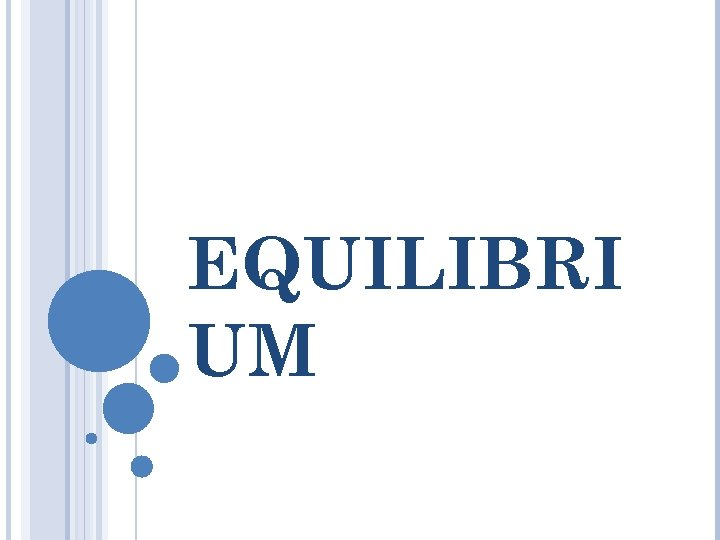EQUILIBRI UM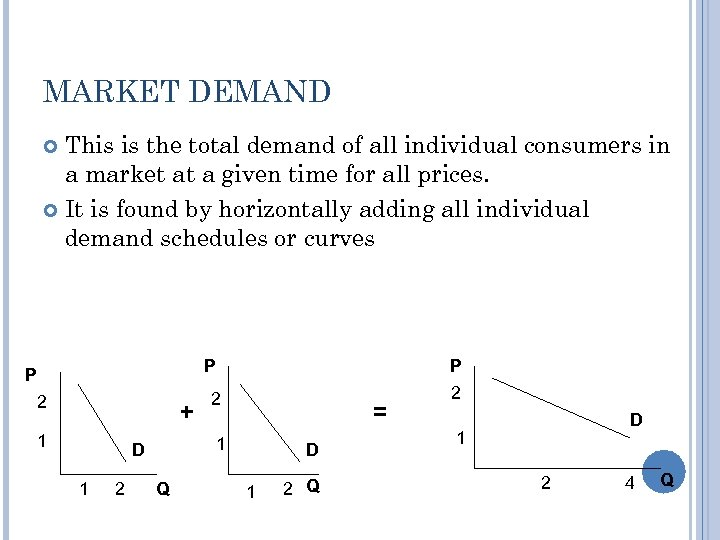MARKET DEMAND This is the total demand of all individual consumers in a market at a given time for all prices. It is found by horizontally adding all individual demand schedules or curves P P 2 + 1 2 2 2 = 1 D 1 P Q D 1 2 4 Q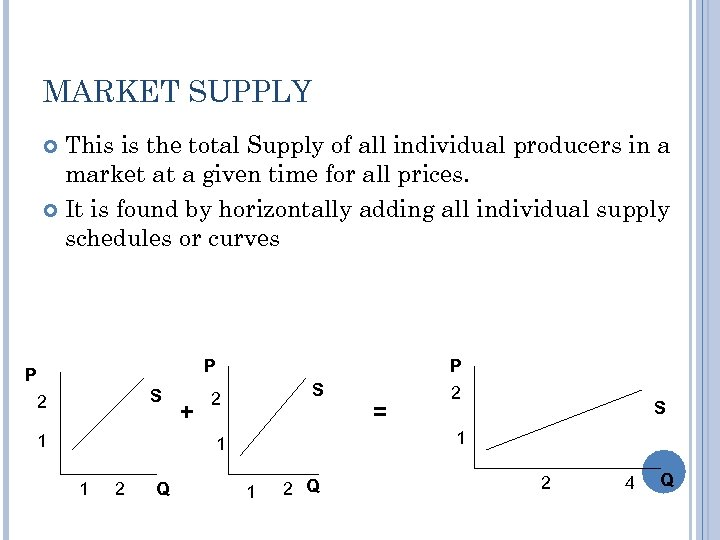MARKET SUPPLY This is the total Supply of all individual producers in a market at a given time for all prices. It is found by horizontally adding all individual supply schedules or curves P P S 2 1 + P S 2 2 Q S 1 1 1 = 2 1 2 Q 2 4 Q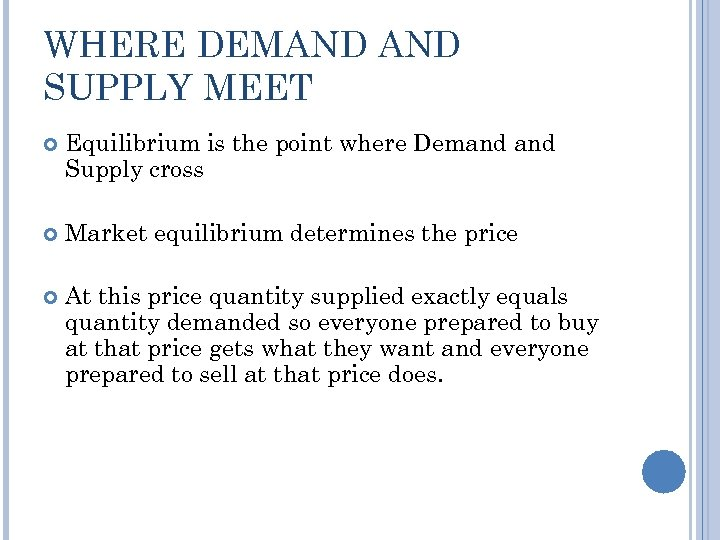WHERE DEMAND SUPPLY MEET Equilibrium is the point where Demand Supply cross Market equilibrium determines the price At this price quantity supplied exactly equals quantity demanded so everyone prepared to buy at that price gets what they want and everyone prepared to sell at that price does.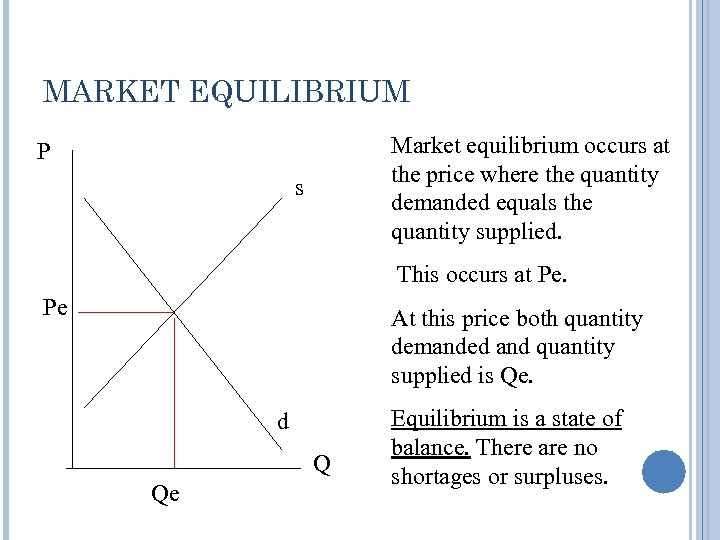MARKET EQUILIBRIUM Market equilibrium occurs at the price where the quantity demanded equals the quantity supplied. P s This occurs at Pe. Pe At this price both quantity demanded and quantity supplied is Qe. d Q Qe Equilibrium is a state of balance. There are no shortages or surpluses.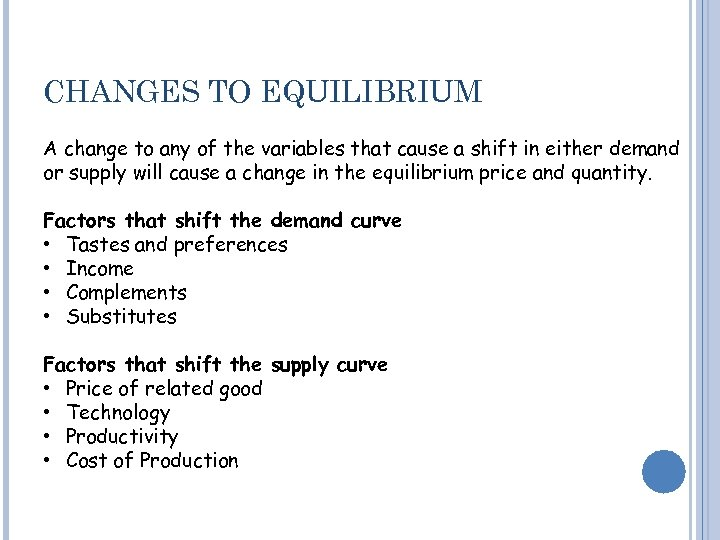CHANGES TO EQUILIBRIUM A change to any of the variables that cause a shift in either demand or supply will cause a change in the equilibrium price and quantity. Factors that shift the demand curve • Tastes and preferences • Income • Complements • Substitutes Factors that shift the supply curve • Price of related good • Technology • Productivity • Cost of Production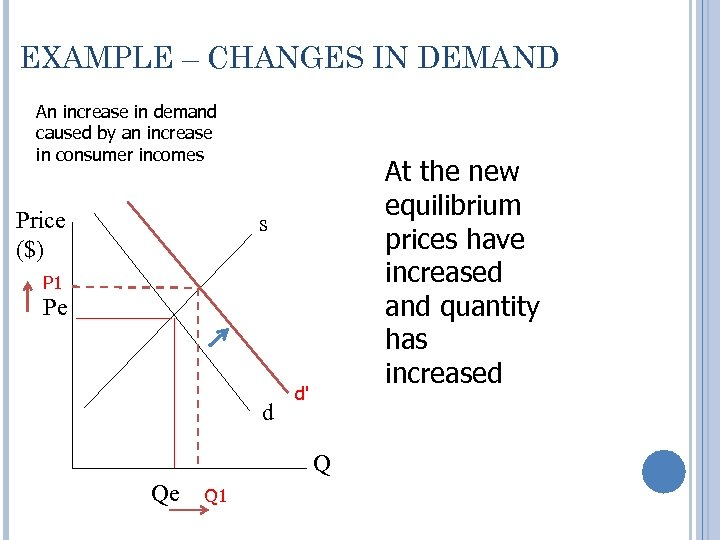EXAMPLE – CHANGES IN DEMAND An increase in demand caused by an increase in consumer incomes Price (\$) At the new equilibrium prices have increased and quantity has increased s P 1 Pe d d' Q Qe Q 1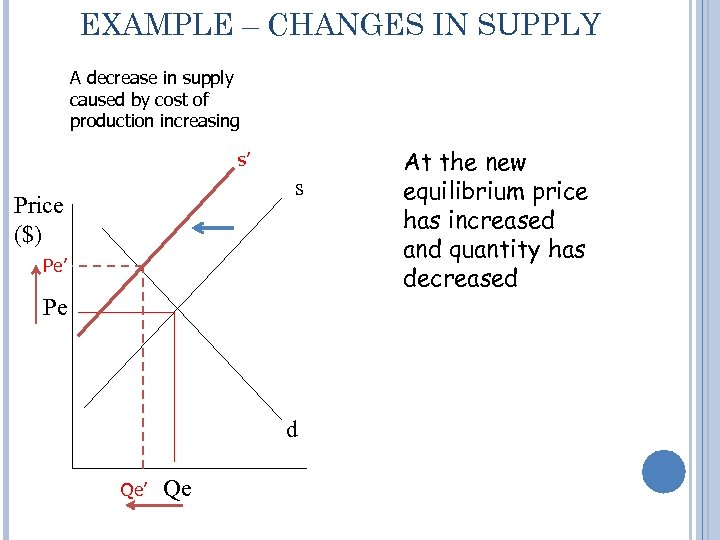EXAMPLE – CHANGES IN SUPPLY A decrease in supply caused by cost of production increasing s’ s Price (\$) Pe’ Pe d Qe’ Qe At the new equilibrium price has increased and quantity has decreased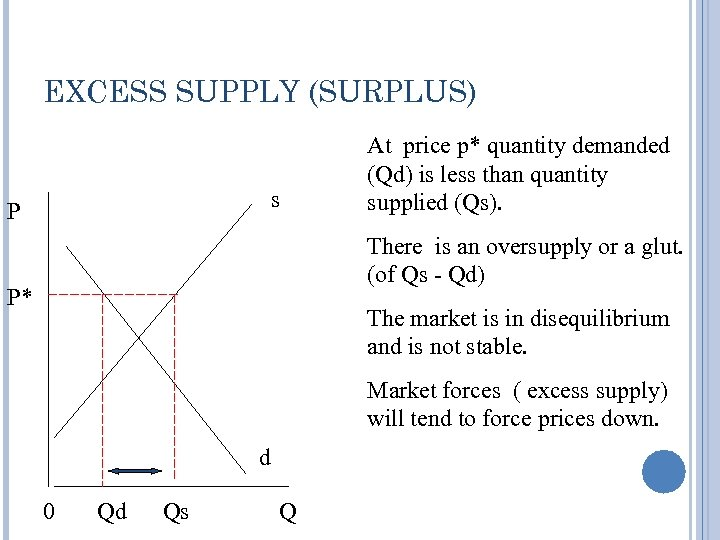EXCESS SUPPLY (SURPLUS) s P At price p* quantity demanded (Qd) is less than quantity supplied (Qs). There is an oversupply or a glut. (of Qs - Qd) P* The market is in disequilibrium and is not stable. Market forces ( excess supply) will tend to force prices down. d 0 Qd Qs Q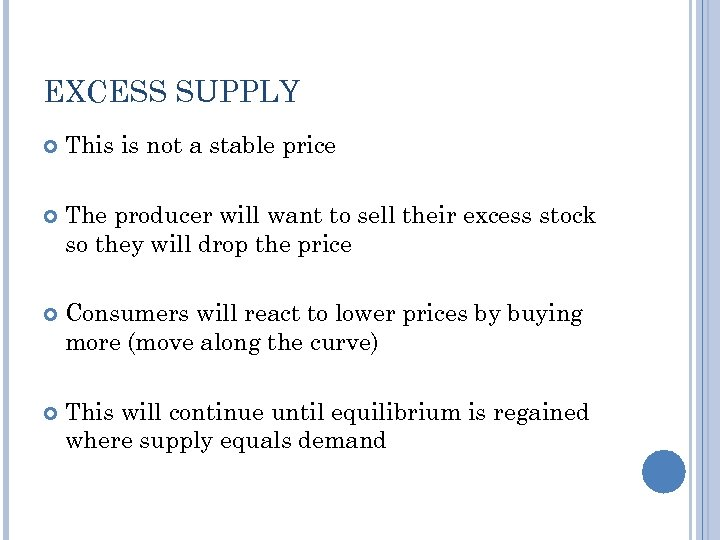EXCESS SUPPLY This is not a stable price The producer will want to sell their excess stock so they will drop the price Consumers will react to lower prices by buying more (move along the curve) This will continue until equilibrium is regained where supply equals demand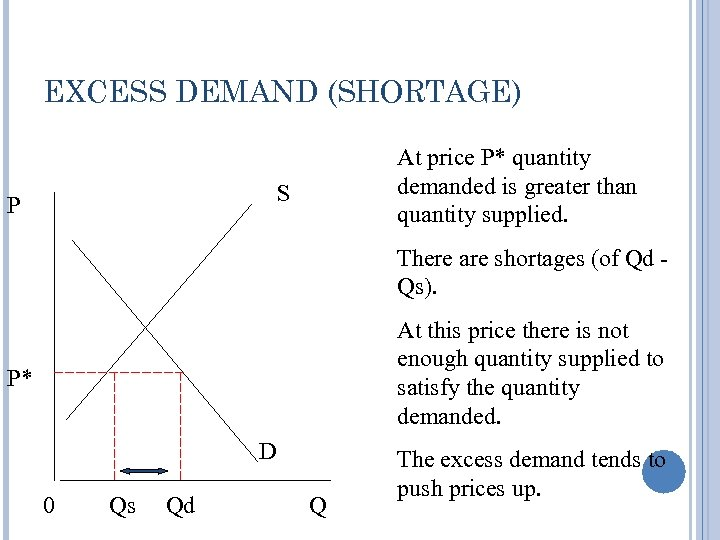EXCESS DEMAND (SHORTAGE) At price P* quantity demanded is greater than quantity supplied. S P There are shortages (of Qd Qs). At this price there is not enough quantity supplied to satisfy the quantity demanded. P* D 0 Qs Qd Q The excess demand tends to push prices up.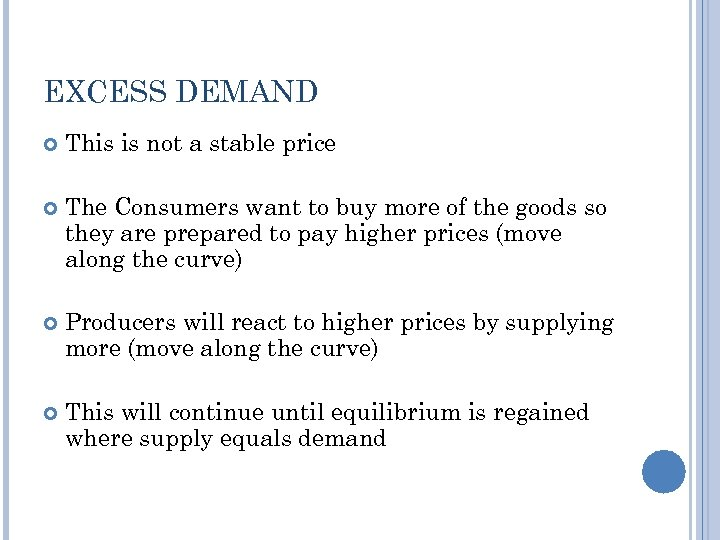EXCESS DEMAND This is not a stable price The Consumers want to buy more of the goods so they are prepared to pay higher prices (move along the curve) Producers will react to higher prices by supplying more (move along the curve) This will continue until equilibrium is regained where supply equals demand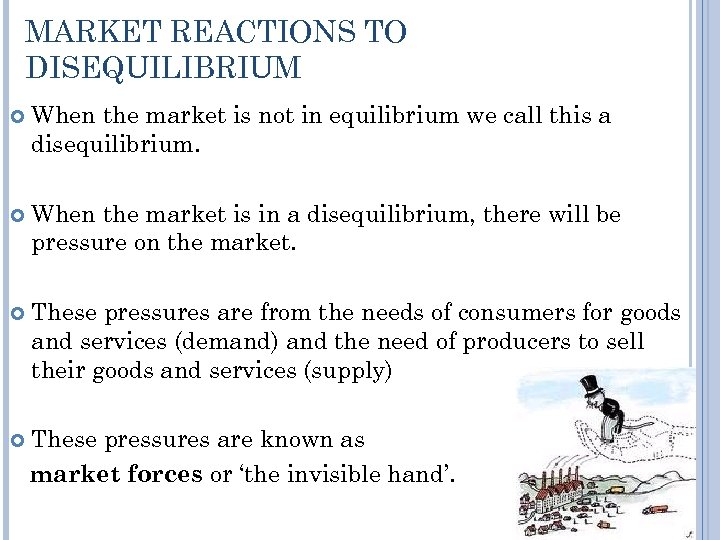MARKET REACTIONS TO DISEQUILIBRIUM When the market is not in equilibrium we call this a disequilibrium. When the market is in a disequilibrium, there will be pressure on the market. These pressures are from the needs of consumers for goods and services (demand) and the need of producers to sell their goods and services (supply) These pressures are known as market forces or ‘the invisible hand’.